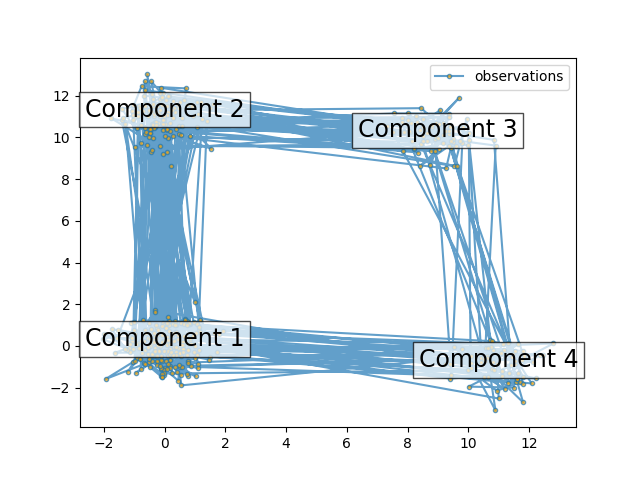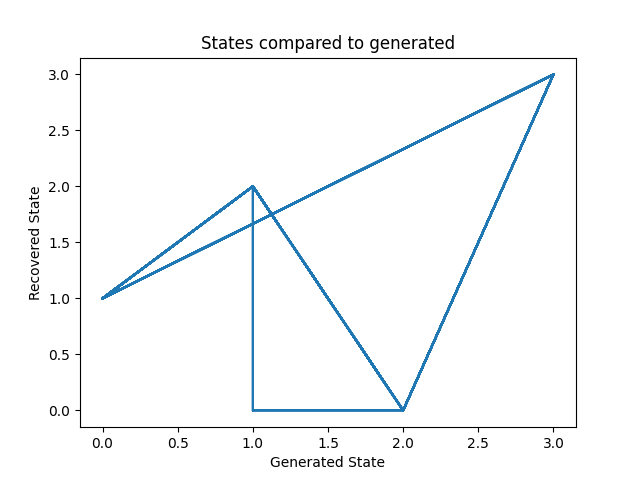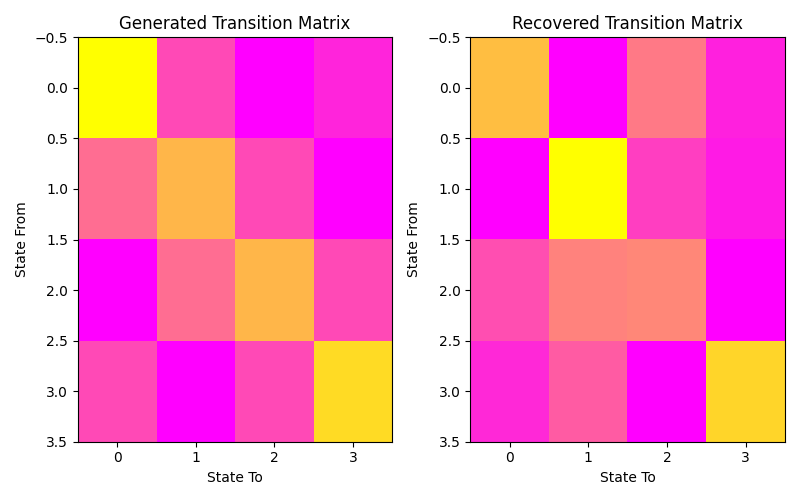# Sampling from and decoding an HMM#

This script shows how to sample points from a Hidden Markov Model (HMM): we use a 4-state model with specified mean and covariance.

The plot shows the sequence of observations generated with the transitions between them. We can see that, as specified by our transition matrix, there are no transition between component 1 and 3.

Then, we decode our model to recover the input parameters.

```import numpy as np
import matplotlib.pyplot as plt

from hmmlearn import hmm

# Prepare parameters for a 4-components HMM
# Initial population probability
startprob = np.array([0.6, 0.3, 0.1, 0.0])
# The transition matrix, note that there are no transitions possible
# between component 1 and 3
transmat = np.array([[0.7, 0.2, 0.0, 0.1],
[0.3, 0.5, 0.2, 0.0],
[0.0, 0.3, 0.5, 0.2],
[0.2, 0.0, 0.2, 0.6]])
# The means of each component
means = np.array([[0.0, 0.0],
[0.0, 11.0],
[9.0, 10.0],
[11.0, -1.0]])
# The covariance of each component
covars = .5 * np.tile(np.identity(2), (4, 1, 1))

# Build an HMM instance and set parameters
gen_model = hmm.GaussianHMM(n_components=4, covariance_type="full")

# Instead of fitting it from the data, we directly set the estimated
# parameters, the means and covariance of the components
gen_model.startprob_ = startprob
gen_model.transmat_ = transmat
gen_model.means_ = means
gen_model.covars_ = covars

# Generate samples
X, Z = gen_model.sample(500)

# Plot the sampled data
fig, ax = plt.subplots()
ax.plot(X[:, 0], X[:, 1], ".-", label="observations", ms=6,
mfc="orange", alpha=0.7)

# Indicate the component numbers
for i, m in enumerate(means):
ax.text(m, m, 'Component %i' % (i + 1),
size=17, horizontalalignment='center',
bbox=dict(alpha=.7, facecolor='w'))
ax.legend(loc='best')
fig.show()
```Now, let’s ensure we can recover our parameters.

```scores = list()
models = list()
for n_components in (3, 4, 5):
for idx in range(10):
# define our hidden Markov model
model = hmm.GaussianHMM(n_components=n_components,
covariance_type='full',
random_state=idx)
model.fit(X[:X.shape // 2])  # 50/50 train/validate
models.append(model)
scores.append(model.score(X[X.shape // 2:]))
print(f'Converged: {model.monitor_.converged}'
f'\tScore: {scores[-1]}')

model = models[np.argmax(scores)]
n_states = model.n_components
print(f'The best model had a score of {max(scores)} and {n_states} '
'states')

# use the Viterbi algorithm to predict the most likely sequence of states
# given the model
states = model.predict(X)
```
```Converged: True Score: -1240.2224657040947
Converged: True Score: -1186.7420418264308
Converged: True Score: -1096.768418968077
Converged: True Score: -1088.7116582720425
Converged: True Score: -1297.5072996673102
Converged: True Score: -1088.7116582720416
Converged: True Score: -1088.7116582720437
Converged: True Score: -1088.7116582720469
Converged: True Score: -1088.7116582720416
Converged: True Score: -1088.7116582720416
Converged: True Score: -928.1431723033875
Converged: True Score: -1254.8692925887642
Converged: True Score: -928.1431723033854
Converged: True Score: -1048.9977316520922
Converged: True Score: -928.143172303387
Converged: True Score: -1100.2301663437845
Converged: True Score: -1018.2289220745046
Converged: True Score: -928.1431723033827
Converged: True Score: -928.1431723033828
Converged: True Score: -927.5166437871019
Converged: True Score: -933.8462101735552
Converged: True Score: -947.5748391403899
Converged: True Score: -979.0591339631765
Converged: True Score: -939.4206042890555
Converged: True Score: -944.3509619126286
Converged: True Score: -1039.8531152832604
Converged: True Score: -926.2876665213995
Converged: True Score: -965.3334333311909
Converged: True Score: -1109.8192587178862
Converged: True Score: -1102.0994146229873
The best model had a score of -926.2876665213995 and 5 states
```

Let’s plot our states compared to those generated and our transition matrix to get a sense of our model. We can see that the recovered states follow the same path as the generated states, just with the identities of the states transposed (i.e. instead of following a square as in the first figure, the nodes are switch around but this does not change the basic pattern). The same is true for the transition matrix.

```# plot model states over time
fig, ax = plt.subplots()
ax.plot(Z, states)
ax.set_title('States compared to generated')
ax.set_xlabel('Generated State')
ax.set_ylabel('Recovered State')
fig.show()

# plot the transition matrix
fig, (ax1, ax2) = plt.subplots(1, 2, figsize=(8, 5))
ax1.imshow(gen_model.transmat_, aspect='auto', cmap='spring')
ax1.set_title('Generated Transition Matrix')
ax2.imshow(model.transmat_, aspect='auto', cmap='spring')
ax2.set_title('Recovered Transition Matrix')
for ax in (ax1, ax2):
ax.set_xlabel('State To')
ax.set_ylabel('State From')

fig.tight_layout()
fig.show()
```
••Total running time of the script: ( 0 minutes 1.663 seconds)

Gallery generated by Sphinx-Gallery